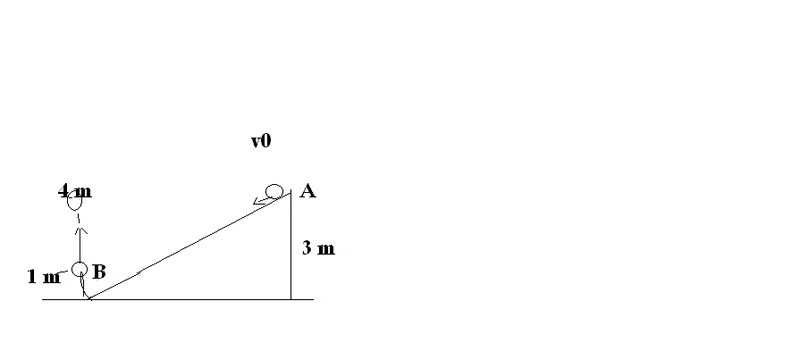# What is the initial speed v0 of the ball?1) What is the initial speed v0 of the ball?

2)What is the height of the ball when the vertical speed is 1/2 of the initial speed vo?

Thanks
(I'm so sorry for my bad english. It's not my mother tongue)

Last edited:

Astronuc
Staff Emeritus
Well, vo is the magnitude of the velocity, and since velocity is a vector, there must be a unit vector associated with that magnitude, however . . .

one must determine the vertical displacement after the ball is deflected up, and actually, I believe the appropriate question is "what is the height of the ball when the vertical speed is 1/2 of the initial speed vo?"

One could use conservation of energy.

Last edited:
We have:

0.5mv^2+mgh = 0.5mv0^2+mgh0

v0^2 = -2mgh0 + mv^2 + 2mgh

v0 = (-2gh0 + v^2 + 2gh)^1/2

v0 = (- 2 * 9.81 * 4 + o m/s + 2 * 9.81 * 3)^1/2

v0 = (- 78 + 58.86)^1/2

Here is something wrong

Astronuc
Staff Emeritus
The initial equation 0.5mv^2+mgh = 0.5mv0^2+mgh0 is incorrect.

One is solving for the h, when v = vo/2, so

0.5 m (vo/2)2 + mgh = 0.5 m vo2 + mgho and m divides out, so

0.5 (vo/2)2 + gh = 0.5 vo2 + gho, and the re-arrange terms to get

h = ho + 1/g ( . . . )

Hi, thanks

Maybe I must edit my first question.

What I meant was:

1) How fast must the initial speed v0 be, if the ball shall reach 4 meters into the air (at the heighest, of course)

Hi

I tried to rearrange your equation:

0.5 (vo/2)2 + gh = 0.5 vo2 + gho

I get:

h = ((v0^2)-(v0^8)+gh0))/(g)

Maybe I am just stupid

Astronuc
Staff Emeritus
In h = ((v0^2)-(v0^8)+gh0))/(g), the kinetic energy terms should be

vo2 - (vo/2)2 =

vo2 - vo2/4 = 3/4 vo2

and lets not forget the 1/2 in front of the v2 terms,

so the equation for h should be

h = 1/2 (3/4 vo2)/g + ho =

3/8 (vo2)/g + ho

To solve for the initial speed v0:

v0^2 = v^2 + 2gh - gh0

v0 = (v^2 + 2gh - gh0)^1/2

v0 = (2g(h-h0))^1/2

v0 = (2 * 9.81 (4 m -3 m))^1/2

v0 = 4.43 m/s

What is wrong?

nrqed
Homework Helper
Gold Member
Spruance said:
To solve for the initial speed v0:

v0^2 = v^2 + 2gh - gh0

v0 = (v^2 + 2gh - gh0)^1/2

v0 = (2g(h-h0))^1/2

v0 = (2 * 9.81 (4 m -3 m))^1/2

v0 = 4.43 m/s

What is wrong?
you forgot a 2 in the first equation but then you put it back later so the answer should be right. How do you know it's not? (if you are using Webassign, it may be simply a question of sig figs)

Ok.

Let us assume that the initial speed is 4.43 m/s

then the height of ball is given:

h = 1/2 (3/4 vo2)/g + ho =

3/8 (vo2)/g + ho

= 3/8 * ((4.43^2)/9.81) + 3

= 3.75 m

Is that correct?

nrqed
Homework Helper
Gold Member
Spruance said:
Ok.

Let us assume that the initial speed is 4.43 m/s

then the height of ball is given:

h = 1/2 (3/4 vo2)/g + ho =

3/8 (vo2)/g + ho

= 3/8 * ((4.43^2)/9.81) + 3

= 3.75 m

Is that correct?
you shoudl have mentioned that you has switched to question 2 now...
Yes, that looks right.